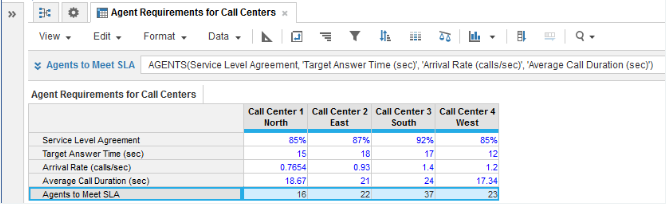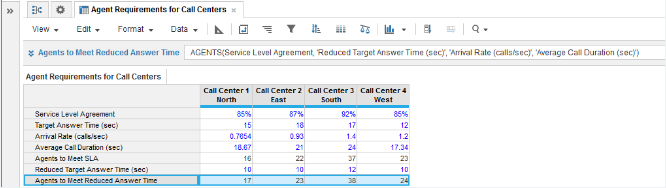1. Calculation functions
2. All Functions
3. Call Center Planning Functions
4. AGENTSCalculates the number of agents required to service a given number of calls and meet the service level agreement.

## Syntax

AGENTS(w, t, y, z)

where:

• w: service level agreement or the percentage of calls to be answered in the target answer time
• y: arrival rate of calls or number of calls per unit of time
• z: average call duration

## Format

Input Format Output Format

w: number (percentage).

t: number(time unit)

y: number

z: number (time unit)

No Data

## Arguments

The function uses the following arguments:

• w: Number - Numeric line item, property, or expression
• t: Number - Numeric line item, property, or expression - Must match the unit as used for arguments y & z
• y: Number - Numeric line item, property, or expression - Must match the unit as used for argument t & z
• z: Number - Numeric line item, property, or expression - Must match the unit as used for argument t & y

## Constraints

The function has the following constraints:

• All parameters are mandatory.
• All parameters must be a numeric line item, property, or expression.
• Time unit used for the t, y, and z parameters must be the same.
• Result line item must be numeric.
• Maximum number of agents is five million.

## Excel equivalent

• No Excel equivalent

## Example

In this example, we have four call centers each covering a separate geographical area:

• Each call center must achieve a service level agreement (SLA), expressed as the percentage of incoming calls that will be answered for a given target answer time.
• The arrival rate of incoming calls and the average call duration are known for each call center from historical monitoring of operational performance.

With the baseline metrics entered for each call center in numeric source line items for Service Level Agreement, Target Answer Time (sec), Arrival Rate (calls/sec), and Average Call Duration (sec), we can use the AGENTS function in an Agents to Meet SLA numeric result line item to calculate how many agents are required for each call center to meet the SLA:Example 2

This second example develops Example 1 to use the AGENTS function to perform what-if analysis for improved answer times you would like to achieve across your call centers. A second Reduced Target Answer Time (sec) source line item is introduced for entering the improved answer times. You can then use a second Agents to Meet Reduced Answer Time result line item for the number of agents needed to reduce call answer times while maintaining the SLA: